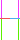# Help with platformer collision

#1

I’ve been working on a 2D raycast-based platformer physics system, and I keep running into the issue of which order to check for collision. If I check for floors then walls, the player will “stand” on the wall for a frame, since it sees it as a floor before the wall sensors get a chance to push the player out. If I check for walls then floors, I have the opposite problem: the wall sensors see the floor and push the player around before the floors sensors can push them out. Here’s a rough layout of the sensors for reference:Green for floor, magenta for ceiling, and red/blue for wall

1 Like

#2

Hmm. I don’t usually mess with raycasts much, but i think the same method for dealing with collision objects in the tutorials would probably work here, if i understand your problem. Hard to know without a code example, but are you trying to “resolve” collisions with the result of each raycast? In the kinematic collision example, because collisons can happen more than once per frame (and multiple rays can collide per frame as well, if you’re standing on ground but also touching a wall), the idea would be to calculate some variable/property (self.correction in the example) for each ray, which would be cumulatively updated for each ray, and then only applied once in update (self.position = self.position + self.correction, go.set_position(self.position).

0 Likes

#3

Oh, also, you would need to reset self.correction at the beginning of each frame in update. Self.correction = vmath.vector3() or similar.

0 Likes

#4

Sorry, I didn’t explain it very well. The rays correct the player’s position perfectly, but they do so at incorrect times. For example, if the player ran into a wall with enough speed, the floor sensors would take priority over the wall sensors, causing the player to “stand” on the wall for a moment before getting pushed out, instead of just being pushed out like they should. I’m looking for a way to ignore/disable sensors when that happens. There doesn’t seem to be any value in the raycast response that could solve this. Here’s the code for the floor sensors:

Code
``````local function floor_sensor(x_off, length, ray_color, pos)
local from = vmath.vector3(pos.x pos.y - x_off, pos.z)
local to = vmath.vector3(pos.x + x_off, pos.y - length, pos.z)
local ray = physics.raycast(from, to, {hash("world")})

local data = {}

if ray ~= nil then
data.found_tile = true
data.x_pos = ray.position.x
data.y_pos = ray.position.y
data.pos = ray.position
to = ray.position
data.new_pos = vmath.vector3(0, length, 0) + ray.position + x_off
data.fraction = ray.fraction
else
data.found_tile = false
end

if game.debug then
msg.post("@render:", "draw_line", { start_point = from, end_point = to, color = color_convert.hex(data.found_tile and ray_color or "000000", 1) } )
end

return data
end

function update(self, dt)
...
local a = floor_sensor(-9, 20, "5af000", pos)
local b = floor_sensor(9, 20, "64ffa2", pos)

if a.found_tile or b.found_tile then
player.grounded = true
if a.found_tile and b.found_tile then
-- Use whichever sensor found the closest tile
if a.fraction <= b.fraction then
pos = a.new_pos
elseif b.fraction < a.fraction then
pos = b.displace
end
elseif a.found_tile then
pos = a.new_pos
elseif b.found_tile then
pos = b.new_pos
end

if not player.state.grounded then
on_land()
end
else
player.grounded = false
end
...
end
``````
0 Likes